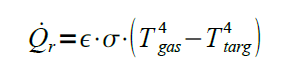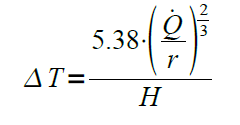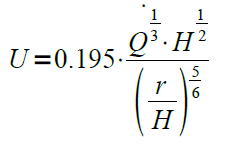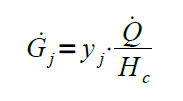# Prediction of fire hazards

Fire is a dynamic process of interacting physics and chemistry, so predicting what is likely to happen under a given set of circumstances is daunting. The simplest predictive methods are algebraic equations. Computer models are used to automate fire hazard calculations and are particularly useful where many repeated calculations must be performed.

## Fire hazard calculations:

Once the design fire curve has been developed, it is then possible to predict the hazards that would result. The types of hazards that might be of interest include the following:

• Radiant heat flux, which affects the potential for ignition of materials and damage to structures or thermal injury to people.
• Smoke production, which dictates the volume of smoke produced.
• Fire plume and ceiling jet temperatures and velocities, which could cause weakening of exposed structural elements.
• Species production, which affects the rate at which an untenable environment could be created.
• Depth of upper layer, which can be used as a surrogate for an untenable environment.

The hazards that are quantified are a function of the objectives of the analysis. For example, if the purpose of the analysis is to determine if a thermally activated detection or suppression system is activated, only the temperatures and velocities of the boom and the ceiling jet can be determined. For the analysis of a smoke control system, only the rate of smoke production can be determined. An evacuation analysis may require the quantification of all listed risks:

Radiant heat flux is a measure of the rate of radiative heat transfer per unit area. An example of radiant heat transfer is the heating that can be felt from exposure to the sun on a hot day, although the intensity of thermal radiation in sunlight is too small to be of concern from a fire standpoint. The radiant heat flux from a single burning item can be predicted as a function of the distance from the item. For radiant heat fluxes resulting from fire gases, such as in a compartment fire, the radiant heat flux can be calculated if the gas temperature and the temperature of the target object are known by applying the following equation,where Q˙ r is the rate of radiant heat transfer (kW), e is the emissivity of gas (0 ≤ e ≤ 1), and s is the Stephan- Boltzmann constant (5.67 × 10–11 kW∙m−2·K−4), Tgas is the temperature of gas (K) and Ttarg is the temperature of target (K).

### Smoke Production:

When calculating smoke production rates, smoke is usually defined as the products of combustion and the air entrained into the fire plume. Therefore, the amount of smoke produced is a function of the height above the fire.

### Fire Plumes and Ceiling Jet Temperatures and Velocities:

A fire will produce a plume of hot gas that will rise and contact the ceiling of a given space (for a compartment or enclosed space), forming a ceiling jet. Similarly, the temperature and velocity of a ceiling jet can be calculated in accordance with the following equations,where ΔT is the temperature rise over ambient (°C), U is the ceiling jet velocity (m∙s−1), H is the height above fire (m), r is the horizontal distance from fire centerline (m), and Q˙ is the total heat release rate (kW).

### Species Production:

Fires can create a number of products of combustion that can be toxic or corrosive, including carbon dioxide (CO2), water vapor (H2O), carbon monoxide (CO), and many others that vary with the fuel and burning conditions. Species production rates can be calculated from the following equation,where G˙ j is the smoke production rate of species j (kg∙s−1), yj is the yield fraction of species j, and ΔHc is the heat of combustion of fuel (kJ∙kg−1).

### Toxicity:

Toxic gases produced by a fire can incapacitate or kill people who are exposed to them. A commonly used approach to determine whether the fire-induced environment is potentially harmful to people exposed is the fractional effective dose (FED) model developed by Purser. This can be expressed as follows,

where FINC is the fraction of an incapacitating dose of all asphyxiating gases, FCO is the fraction of an incapacitating dose of CO, FHCN is the fraction of an incapacitating dose of HCN, FIRR is the fraction of irritant dose, VHYP is the multiplication factor for carbon doixide (CO2) induced hyperventilation, FLOX is the fraction of an incapacitating dose of low-oxygen hypoxia, FIDC is the fraction of an incapacitating dose of CO2.